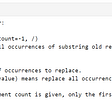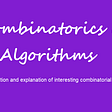# Fractals and the Chaos Game

While looking for some inspiration on how to increase focus and engagement of my Python students (beginners to intermediate preliminary school children), I was looking for some interesting graphical examples on how algorithms works. Needless to say, the example needs to be simple enough to be explained to preliminary school children around age 13 and graphic part is needed as this is really something that works best with young peoples minds (and not only young :) )

This is how I come across the Chaos Game, an example of how simple algorithm can create beautiful shapes if enough iterations are used.

Below short article contains 3 parts:

1. Definition and examples of fractals
2. Fractals in nature
3. The Chaos Game

# 1. Definition and examples of fractals

According to Wikipedia Fractal is a self-similar subset of Euclidean space whose fractal dimension strictly exceeds its topological dimension. Fractals appear the same at different levels.

In essence this means that each part of the fractal is similar to it’s entire shape. It is best described by example.

Koch snowflake
The Koch snowflake can be built up from an equilateral triangle, and each next stage is formed by taking out exact 1/3 of a middle part of triangle’s side and replace it with two lines of exact 1/3 length with 60 degree angle.

Another example of fractal is Sierpinski Triangle with the overall shape of an equilateral triangle, subdivided recursively into smaller equilateral triangles. Below you can see iterations 1 through 5.

There are many more and some of them much more complicated examples of fractals including Mandelbrot set. I encourage you to see for yourself searching for more examples.

# 2. Fractals in nature

Those mathematical “self-similar subset of Euclidean space” are not just theoretical concepts that lives in mathematics equations. Turned out that nature is all about fractals. Broccoli is one big fractal like structure where smaller part of it are much like entire vegetable:

Below block of plexiglass was exposed to a strong current of electricity that burned a fractal branching pattern within. This can be best thought of as bottled-lightning.

Fern is another example of fractal like shape:

There are many more, and again as in the previous section I encourage you to search for yourself to find out more on this interesting topic.

# 3. The Chaos Game

In this game, the fractal is created by iteratively creating a sequence of points, starting with the initial random point, in which each point in the sequence is a given fraction of the distance between the previous point and one of the vertices.

Here is how it works in practice, creating Sierpinski triangle:

1. Create equilateral triangle and mark position of each vertex (in red below)
2. Choose random point within the triangle and mark a dot
3. Choose one of the 3 vertexes of the triangle at random
4. Calculate distance to the chosen vertex and divide by 2
5. Move towards vertex selected in point 3 by distance calculated in point 4
6. Make a dot
7. Repeat from point 3

Here is what is created after few iterations:

When I show this to the students and explain how simply achieve this using Python code they start applying this algorithm to other figures like squares, pentagons etc. trying to find what happens. One of the students come up with hexagon shape with additional rule that in the next iteration algorithm can not choose the same vertex as in the previous iterations. This is what shows up:

Looks like snow flake, isn’t it ?

If you want to check for yourself many other Chaos Game example base on square and other figures, check out Wikipedia.

--

--

--

## More from Analytics Vidhya

Analytics Vidhya is a community of Analytics and Data Science professionals. We are building the next-gen data science ecosystem https://www.analyticsvidhya.com

## MixMarvel Project Details## Documenting the journey from Line Cook to Full Stack Web Developer## Setting up Mysql server for remote connection on linux## Real-Time Media HW 2.2## String Manipulation [Python]## An Optimization Algorithm for a Packing Problem.## Testing your Terraform project on CircleCI## Delightful Designs: Airflow’s Configuration Parser## Using AI to Identify Ingredients and Suggest Recipes